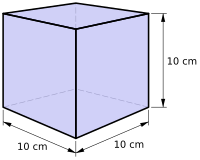# Litre facts for kids

Kids Encyclopedia Facts
(Redirected from Milliliter)One litre has the same volume as this cube. Each side is 10 cm
1 litre of water = 1 kilogram of water (at 4 °C)

A litre (liter in the United States) is one of the metric units of volume. It is not an SI unit.

One litre is the volume of one cubic decimetre, that is a cube of 10 x 10 x 10 centimetres. One litre of water has the mass of exactly one kilogram. This results from the definition given in 1795, where the gram was defined as the weight of one cubic centimetre of melting ice.

The symbol for litre is "L" or "l".

For smaller volumes the decilitre is used: 10 dl = one litre.

For smaller volumes the centilitre is used: 100 cl = one litre.

For smaller volumes the millilitre is used: 1000 ml = one litre.

The capital letter "L" is preferred by some people as the small "l" can look like the number one "1".

• 1 imperial gallon = 4.5461 litres
• 1 US gallon = 3.7854 litres
• 1 litre = 1 dm3

## History

The metric system was first introduced in France in 1791. That system did not have its own unit of capacity or volume because volume can be measured in cubic metres. In 1793 work to make the metric system compulsory in France was started by the Temporary Commission of Republican Weights and Measures. Due to public demand, the commission said that the cubic metre was too big for everyday use. They said that a new unit based on the old cadil should be used instead. One cadil was to be 0.001 cubic metres. This was equivalent to a cube with sides 10 cm. The cadil was also known as the pinte or the litron. The pinte had been an old French unit of measure of capacity. In 1795 the definition was revised. The cadil was given the name litre.

In 1795 the kilogram was defined to be exactly one litre of water at 4 °C. In 1799 the kilogram was redefined. The new definition said that the kilogram was the mass of the kilogram des archives. In 1901 scientists measured the volume of one litre of water at 4 °C very carefully. They found that it occupied about 1.000028 dm3. The BIPM redefined the litre as being exactly the volume of one kilogram of water at 4 °C.

In 1960 the SI was introduced. The BIPM changed the definition of the litre back to "one dm3". The litre is not part of SI. The BIPM defined the litre as a "Non-SI unit accepted for use with the SI". This was because it is used in many countries. The BIPM said that the litre should not be used very very accurate work.

According to SI rules, the symbol for the litre should be "l". This is because the litre was not named after somebody whose name was "Litre". However the symbol "l" and the number "1" are easily confused. In 1979 the BIPM made an exception for the symbol for the litre. They said that people could use either "L" or "l" as its symbol.Litre Facts for Kids. Kiddle Encyclopedia.